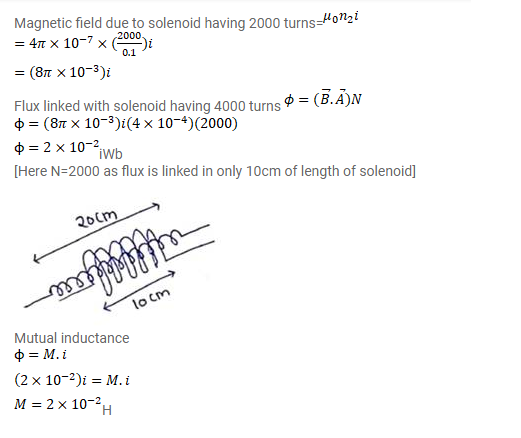# A solenoid of length 20cm,

Question:

A solenoid of length $20 \mathrm{~cm}$, area of cross-section $4.0 \mathrm{~cm}^{2}$ and having 4000 turns is placed inside another solenoid of 2000 turns having a cross-sectional area $8.0 \mathrm{~cm}^{2}$ and length $10 \mathrm{~cm}$. Find the mutual inductance between the solenoids.

Solution: